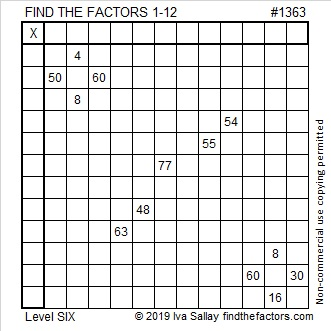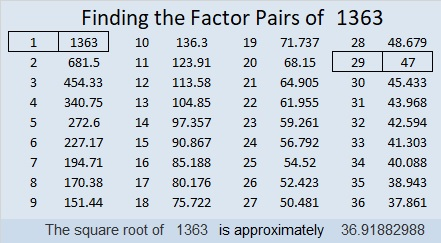1363 and Level 6

The common factors of 60 and 30 allowed in the puzzle are 5, 6, and 10. Which one is the logical choice? Look at the other clues in the puzzle and you should be able to eliminate two of the choices.Print the puzzles or type the solution in this excel file: 12 Factors 1357-1365

Here are a few thoughts about the puzzle number, 1363:

• 1363 is a composite number.
• Prime factorization: 1363 = 29 × 47
• 1363 has no exponents greater than 1 in its prime factorization, so √1363 cannot be simplified.
• The exponents in the prime factorization are 1, and 1. Adding one to each exponent and multiplying we get (1 + 1)(1 + 1) = 2 × 2 = 4. Therefore 1363 has exactly 4 factors.
• The factors of 1363 are outlined with their factor pair partners in the graphic below.1363 is the hypotenuse of a Pythagorean triple:
940-987-1363 which is (20-21-29) times 47

This site uses Akismet to reduce spam. Learn how your comment data is processed.